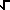# Math2.org Math Tables: Complexity(Math)

### Rationale that( i ) =(1/2) + i(1/2)

Assuming that( i ) =(1/2) + i(1/2)
we can square both sides to get
i = [(1/2) + i(1/2) ]2
i = [ (1/2) + 2(1/2) i + (1/2) i2 ]
i = [ (1/2) + i + (1/2) (-1) ]
i = i (which is a true statement)

This is not a proof, but simply evidence that the formula is correct.